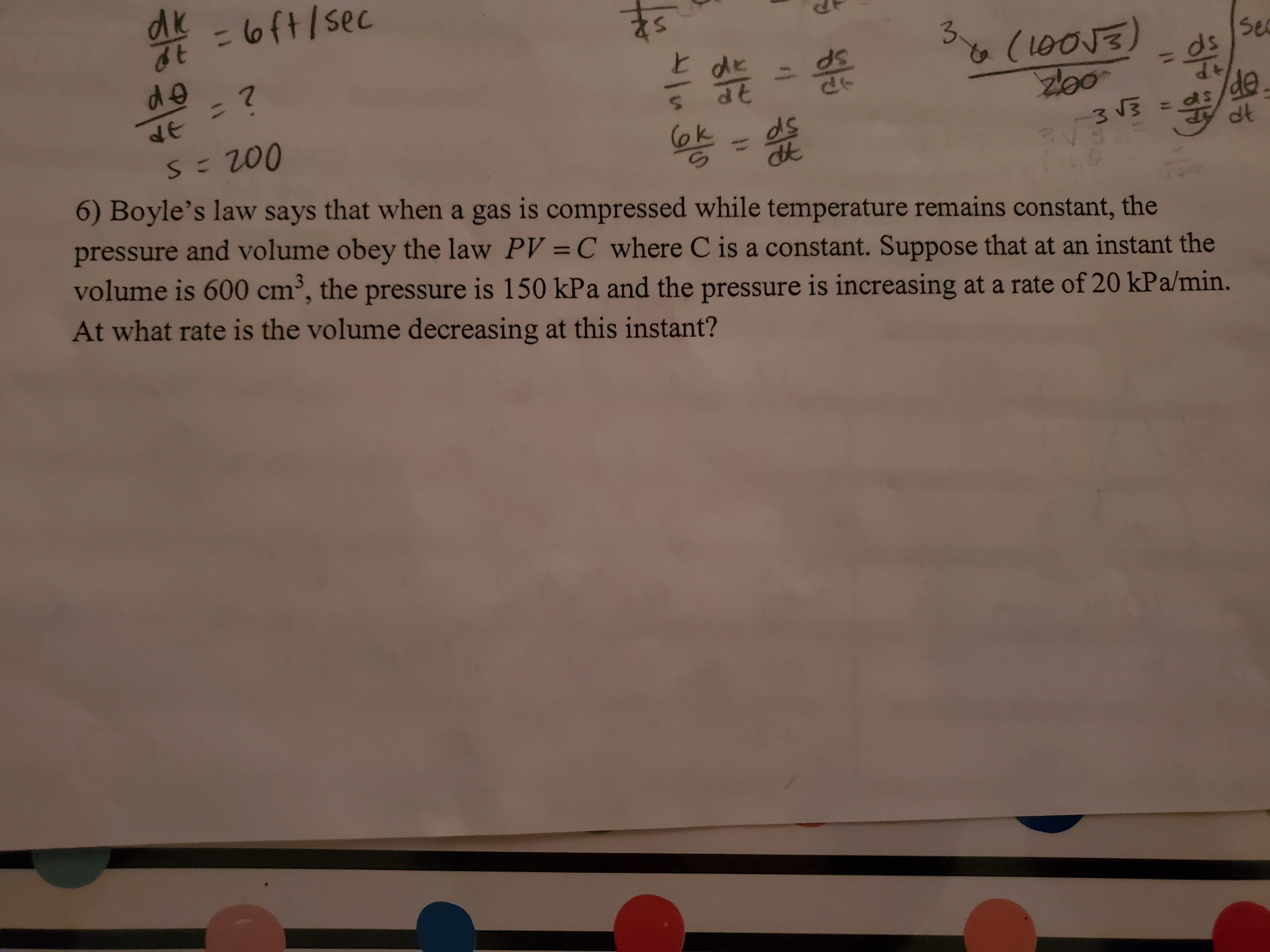# - 1oft/secE deSefe=?S 200+PdeCok3 V36) Boyle's law says that when a gas is compressed while temperature remains constant, thepressure and volume obey the law PV = C where C is a constant. Suppose that at an instant thevolume is 600 cm, the pressure is 150 kPa and the pressure is increasing at a rate of 20 kPa/min.At what rate is the volume decreasing at this instant?

Question
11 views

Related rateshelp_outlineImage Transcriptionclose- 1 oft/sec E de Se fe=? S 200 +P de Cok 3 V3 6) Boyle's law says that when a gas is compressed while temperature remains constant, the pressure and volume obey the law PV = C where C is a constant. Suppose that at an instant the volume is 600 cm, the pressure is 150 kPa and the pressure is increasing at a rate of 20 kPa/min. At what rate is the volume decreasing at this instant? fullscreen
check_circle

Step 1

Given in the question that

Step 2

Differentiate with respect to t,

Step 3

Given tha...

### Want to see the full answer?

See Solution

#### Want to see this answer and more?

Solutions are written by subject experts who are available 24/7. Questions are typically answered within 1 hour.*

See Solution
*Response times may vary by subject and question.
Tagged in

### Physics